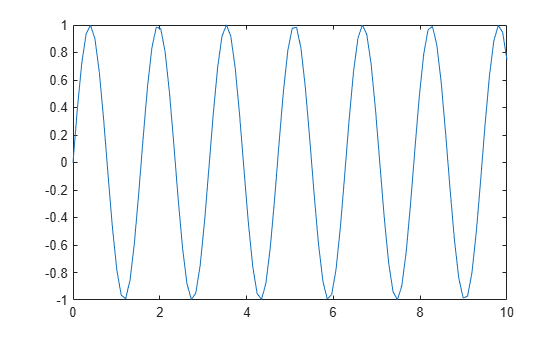Documentation

### This is machine translation

Mouseover text to see original. Click the button below to return to the English version of the page.

Note: This page has been translated by MathWorks. Click here to see
To view all translated materials including this page, select Country from the country navigator on the bottom of this page.

# gca

Current axes or chart

## Syntax

``ax = gca``

## Description

example

````ax = gca` returns the current axes or chart for the current figure, which is typically the last one created or clicked with the mouse. Graphics functions, such as `title`, target the current axes or chart. Use `ax` to access and modify properties of the axes or chart. If axes or charts do not exist, then `gca` creates Cartesian axes.```

## Examples

collapse all

Plot a sine wave.

```x = linspace(0,10); y = sin(4*x); plot(x,y)```Set the font size, tick direction, tick length, and y-axis limits for the current axes. Use `gca` to refer to the current axes.

Note: Starting in R2014b, you can use dot notation to set properties. If you are using an earlier release, use the `set` function instead, such as `set(ax,'FontSize',12)`.

```ax = gca; % current axes ax.FontSize = 12; ax.TickDir = 'out'; ax.TickLength = [0.02 0.02]; ax.YLim = [-2 2];```## Output Arguments

collapse all

Current axes or chart, returned as an `Axes` object, a `PolarAxes` object, or a graphics object whose parent is a `Figure`, `Tab`, or `Panel` object, instead of an `Axes` object.

For example, a `HeatmapChart` object can be the current chart since the parent is typically a `Figure` object. A `Stem` object cannot be the current chart since the parent is an `Axes` object.

## Tips

• User interaction can change the current axes or chart. It is better to assign the axes or chart to a variable when you create it instead of relying on `gca`.

• Changing the current figure also changes the current axes or chart.

• Set axes properties after plotting since some plotting functions reset axes properties.

• To access the current axes or chart without forcing the creation of Cartesian axes, query the figure `CurrentAxes` property. MATLAB® returns an empty array if there is no current axes.

```fig = gcf; ax = fig.CurrentAxes;```
Starting in R2014b, you can query properties using dot notation. If you are using an earlier release, use the `get` function instead, such as `ax = get(fig,'CurrentAxes')`.

Download ebook